For websites and platforms that provide users with content or reviews, it’s important to include a star rating. Recently, I needed to implement a star rating component for a project and the requirements needed were as below:

• Performant (Doesn’t include images)
• Resizable
• Accessible
• Partial star (e.g: 3.5 or 3.2) dynamically
• Easy to maintain with CSS

I thought about giving SVG a try, and I wasn’t disappointed. In this article, I will go through the solution and how it works for different scenarios.

## Introduction

Before we dive in, I want to put you in context and show you the requirements that I’m looking for, and all the cases that we need to account for.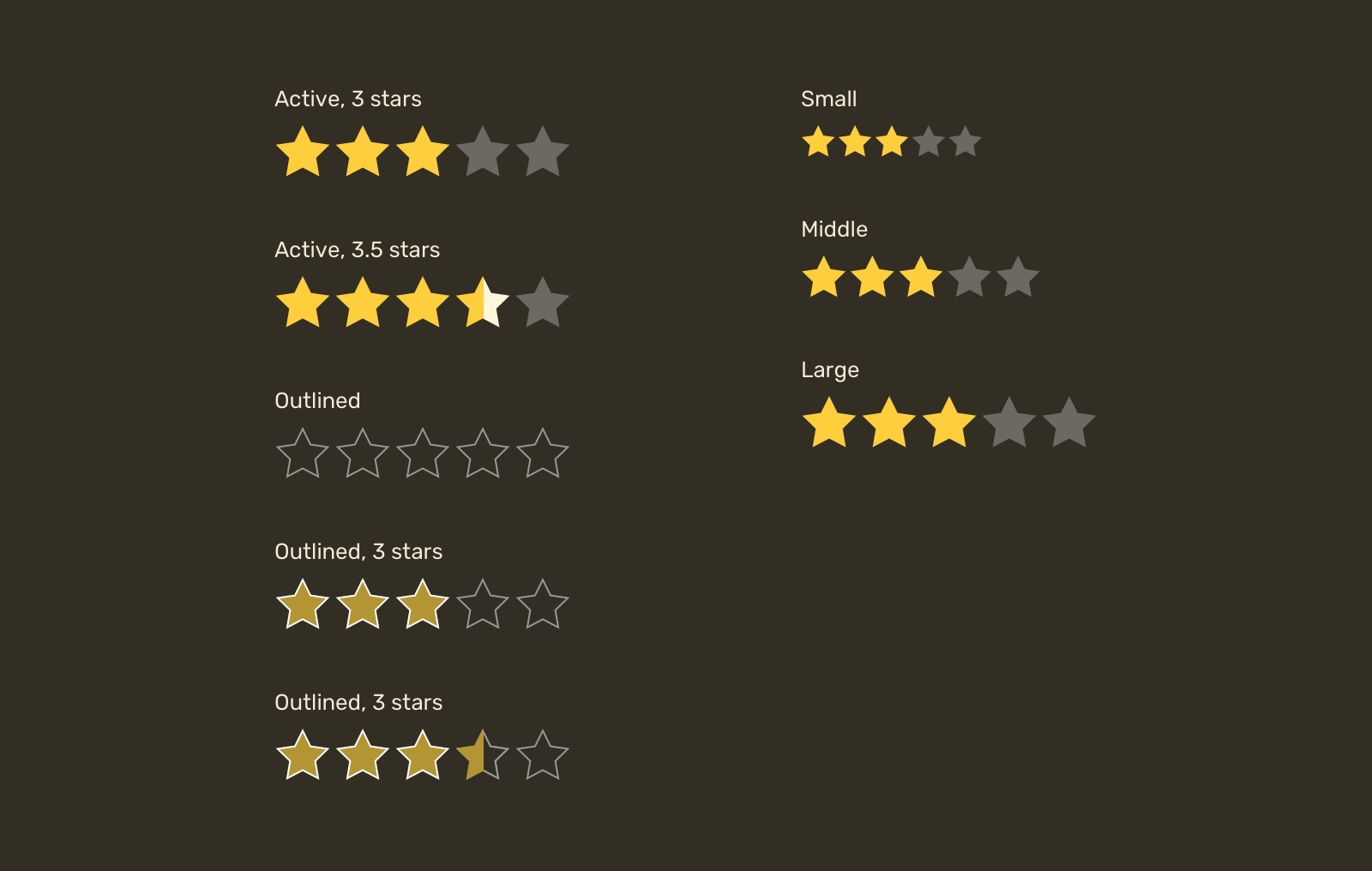Our focus will be on making a star that can be customized by changing its fill or stroke, sizing it, and the ability to show a half star.

Let’s dive in.

## The basic markup

First, you need to pick a star shape and save it as an SVG so we can use it in the browser.

``````<svg
width="32"
height="32"
xmlns="http://www.w3.org/2000/svg"
viewBox="0 0 32 32"
>
<path d="..." />
</svg>``````

In the browser, this will render a black star with a size of `32px` for width and height. Sounds good.

## Accessibility

Keep in mind that you need to include a written rating with an `aria-label` attribute so users with screen readers can access this information.

``````<p aria-label="Rating is 4.5 out of 5">
<svg
width="32"
height="32"
xmlns="http://www.w3.org/2000/svg"
viewBox="0 0 32 32"
>
<path d="..." />
</svg>
</p>``````

## How to reuse the SVG

We can either copy the SVG markup above five times, or extract the path data and save it somewhere, and then reusing it without code duplication. Let’s do that!

First, we need to create an SVG with zero width and height, so it won’t reserve a space.

``````<svg
width="0"
height="0"
xmlns="http://www.w3.org/2000/svg"
>
<!-- Content -->
</svg>``````

Within that SVG, we need to include the path data within a `<symbol>` element. According to MDN:

The symbol element is used to define graphical template objects which can be instantiated by a `<use>` element.

What’s goes inside the `<symbol>` is the same content for the icon. Also, it’s important to add an `id` so we can reference that symbol later.

``````<svg width="0" height="0" xmlns="http://www.w3.org/2000/svg">
<symbol
xmlns="http://www.w3.org/2000/svg"
viewBox="0 0 32 32"
id="star"
>
<path d="..." />
</symbol>
</svg>``````

With that setup, we can now reuse the star symbol with the `<use>` element. The idea is that you need to use the `id` as a value of `href` attribute.

``````<p class="c-rate">
<svg class="c-icon" width="32" height="32">
<use href="#star"></use>
</svg>
<svg class="c-icon" width="32" height="32">
<use href="#star"></use>
</svg>
<svg class="c-icon" width="32" height="32">
<use href="#star"></use>
</svg>
<svg class="c-icon" width="32" height="32">
<use href="#star"></use>
</svg>
<svg class="c-icon" width="32" height="32">
<use href="#star"></use>
</svg>
</p>``````

## Styling the star

Now that we have a list of stars, let’s get into the CSS part. I defined the yellow and grey colors for the star.

``````<p class="c-rate">
<svg class="c-icon active" width="32" height="32">
<use href="#star"></use>
</svg>
<svg class="c-icon active" width="32" height="32">
<use href="#star"></use>
</svg>
<svg class="c-icon active" width="32" height="32">
<use href="#star"></use>
</svg>
<svg class="c-icon active" width="32" height="32">
<use href="#star"></use>
</svg>
<svg class="c-icon" width="32" height="32">
<use href="#star"></use>
</svg>
</p>``````
``````.c-icon {
--star-active: #fece3c;
--star-inactive: #6c6962;
fill: var(--star-inactive);
}

.c-icon.active {
fill: var(--star-active);
}``````

Considering the above markup and styles, the result will be something like the following.## Partial rating

Thankfully, we have two great solutions when it comes to SVG. The first one is using `<masks>` and the second one is with SVG gradients.

### Half star with SVG masks

The goal of using masks is to emulate the effect of erasing a part of the star and painting the other part with a translucent color. To do that, we need the help of SVG masks.In the figure above, we have a square and a star. What we want is the intersection of them, which will result in half a star.

Here is how to do that with SVG:

1. Create a reusable SVG template.
2. Add a `<mask>` element with a rectangle positioned at `x=50%`.
3. Apply the mask to the star shape.
``````<!-- The reusable SVG template -->
<svg viewBox="0 0 32 32" id="star">
<defs>
<rect x="50%" y="0" width="32" height="32" fill="white" />
<symbol viewBox="0 0 32 32" id="star">
<path d="..." />
</symbol>
</defs>
</svg>

<!-- Example of a usage -->
<svg class="c-icon" width="32" height="32" viewBox="0 0 32 32">
</svg>``````

The result will be half a star, just like in the previous figure.

The question is, how we can show a translucent star when it’s being masked? Well, thanks to SVG, we can include multiple elements in the `<mask>`.

With another element, the SVG `<mask>` will look like this:

``````<mask id="half">
<rect x="0" y="0" width="32" height="32" fill="white" />
<rect x="50%" y="0" width="32" height="32" fill="black" />

In masks, a white element represents what we want to show, and a black element represents what we want to hide. When combined, we can create a cut-out effect.

Consider the following figure that explains each element of the mask in a visual way.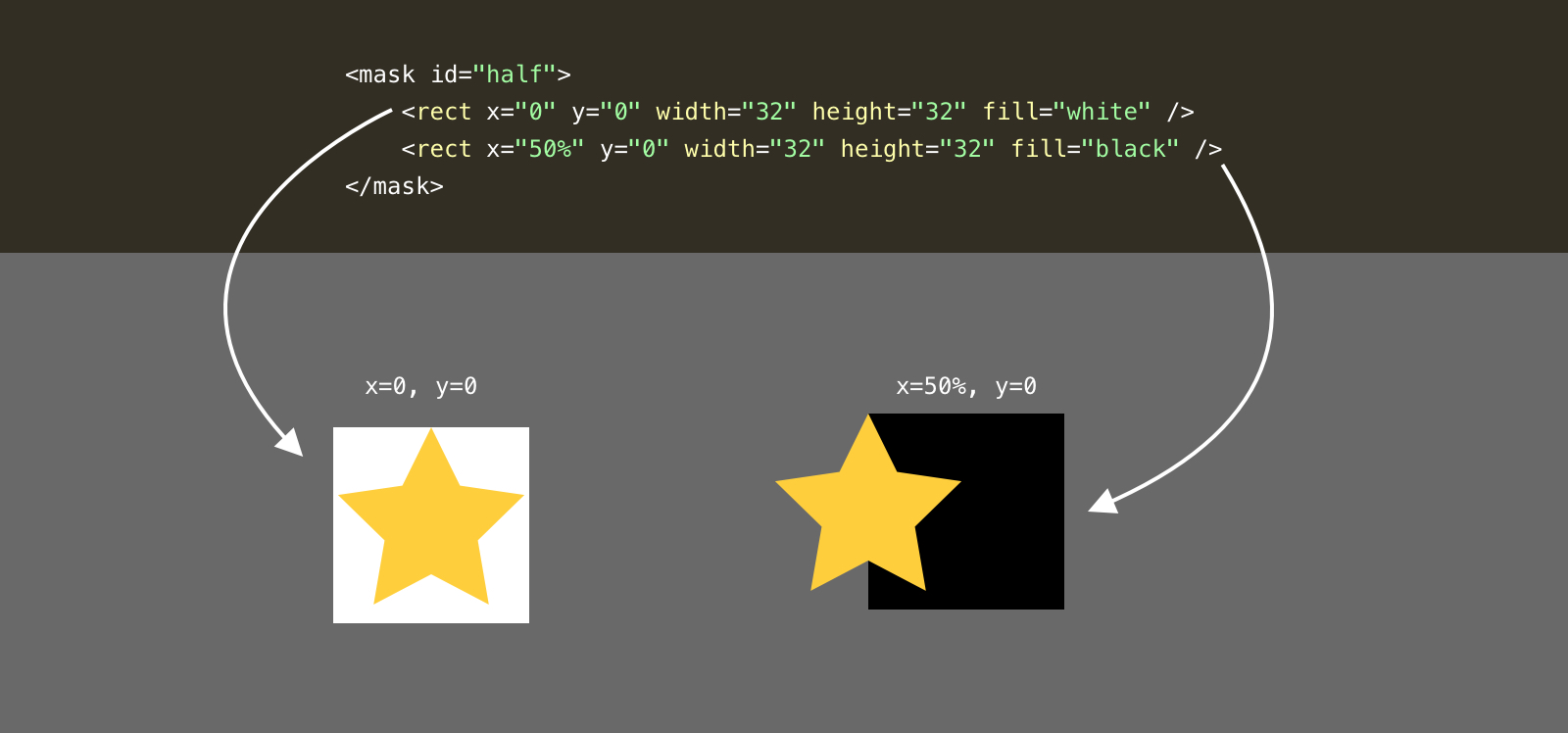Notice that the white rectangle is positioned at the `0,0` point, while the black one is positioned at `50%,0`. Here is how it look visually: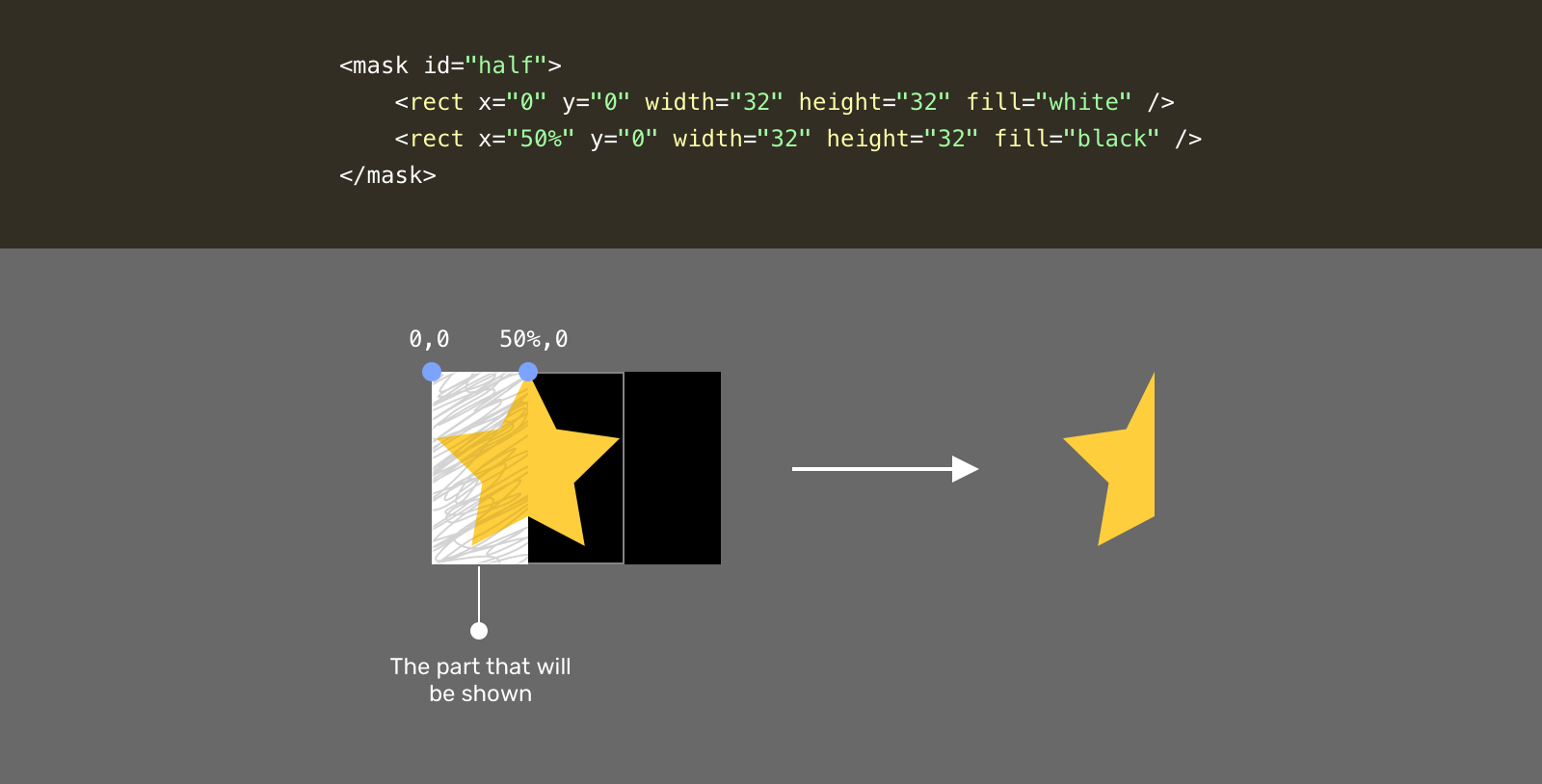Do you see what happened? The part that is scribbled represents the final result, which is half a star. Now, you might be thinking about, how we can add another translucent star to make it more clear?

Well, by using a lighter color than pure black, we will get a translucent effect. That means the area that is currently hidden will have a light shade of the star color.

``````<mask id="half">
<rect x="0" y="0" width="32" height="32" fill="white" />
<rect x="50%" y="0" width="32" height="32" fill="grey" />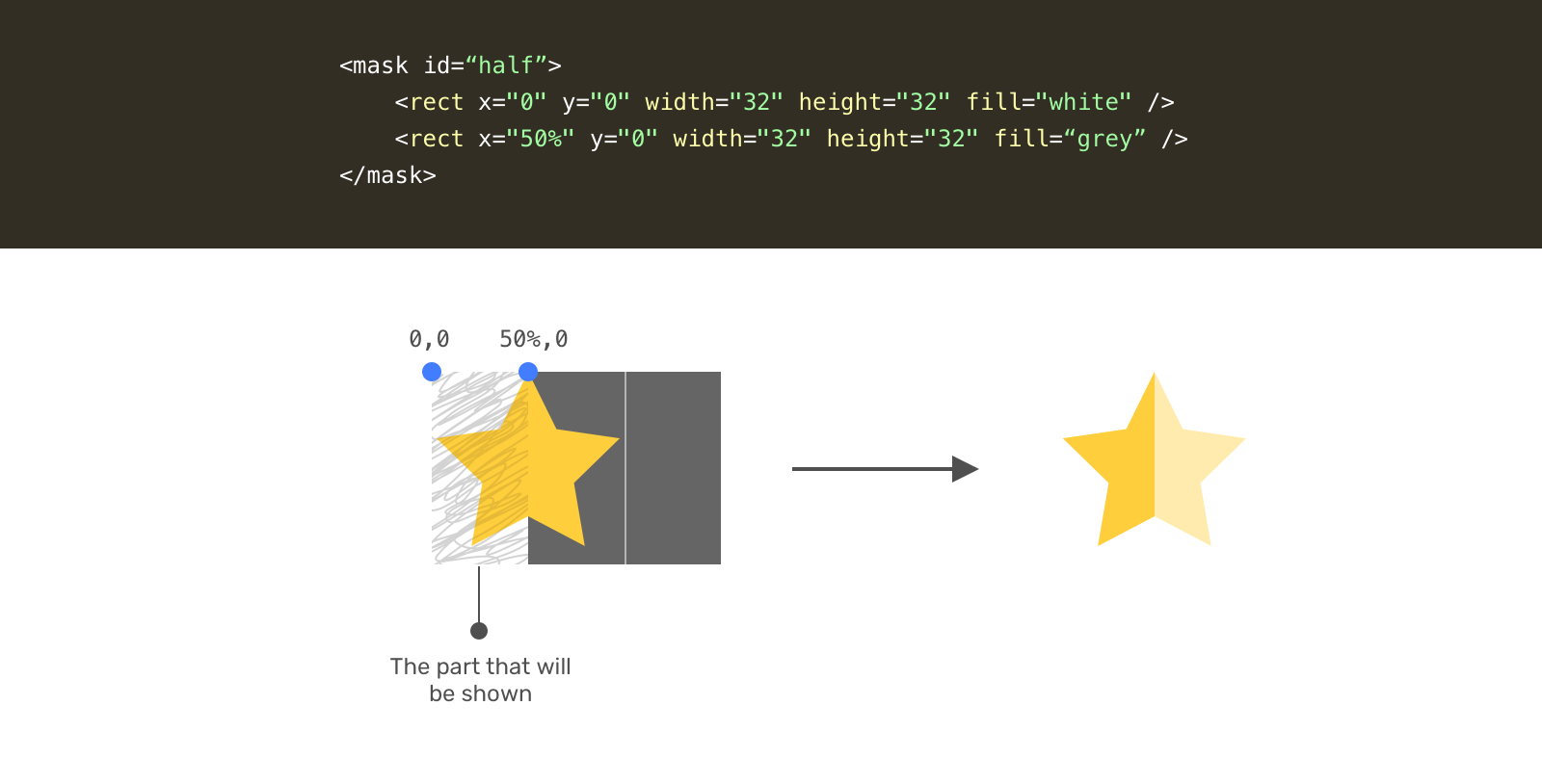Let’s recap with the full SVG markup.

``````<svg style="width: 0; height: 0;" viewBox="0 0 32 32">
<defs>
<rect x="0" y="0" width="32" height="32" fill="white" />
<rect x="50%" y="0" width="32" height="32" fill="grey" />

<symbol viewBox="0 0 32 32" id="star">
<path d="..." />
</symbol>
</defs>
</svg>

<p class="c-rate">
<svg class="c-icon" width="32" height="32" viewBox="0 0 32 32">
</svg>
<!-- 4 more stars -->
</p>``````

With that, we have a star with a partial fill. What’s awesome with that solution is that we don’t need to provide two shades of color. The mask will do the work.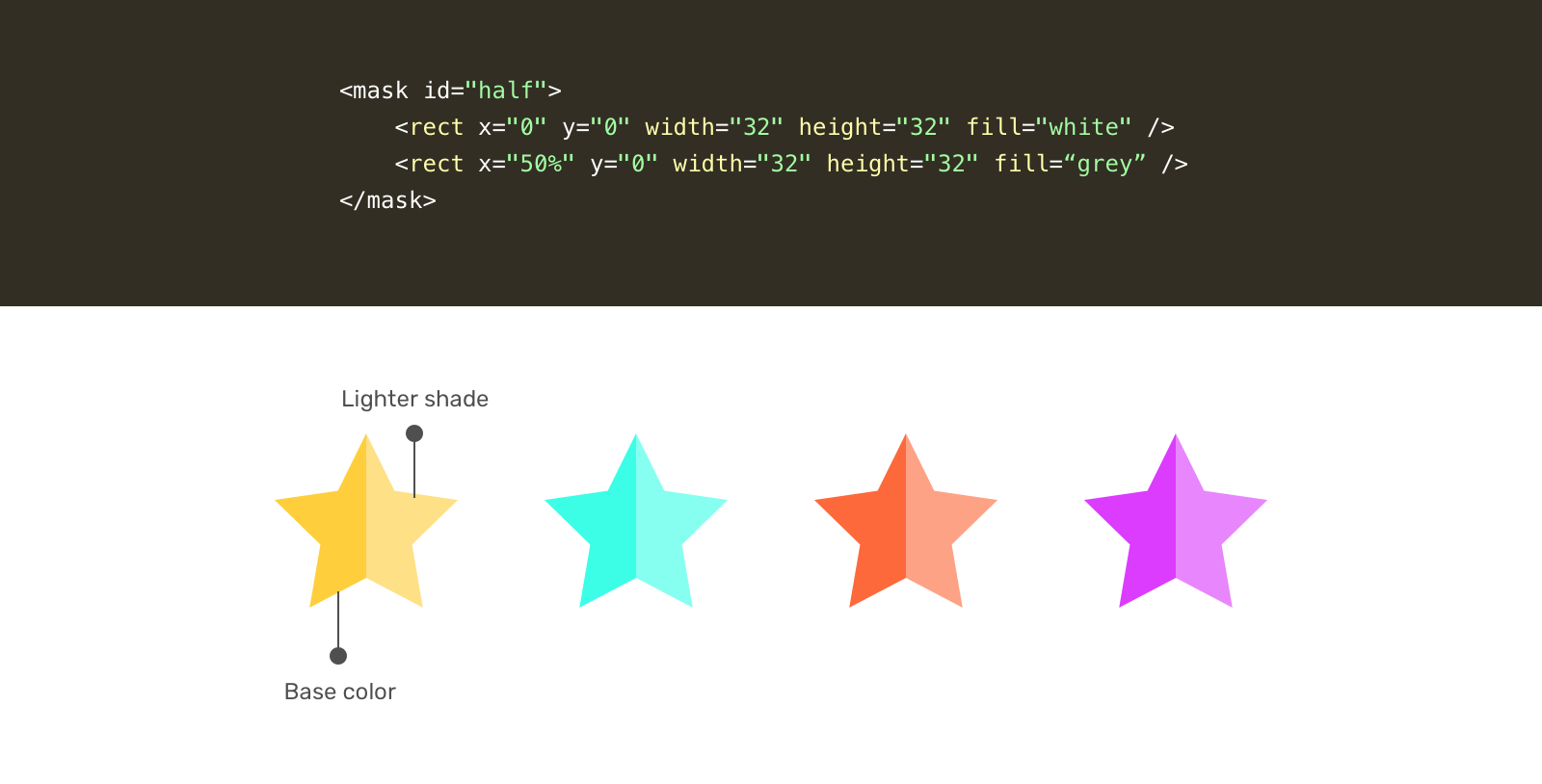Demo

### Half star with SVG gradients

Let’s get into the second solution for a partial star. While searching, I got inspired by this answer on Stackoverflow.

Similar to the mask, we need to define a gradient in the `<defs>` element.

``````<svg style="width: 0; height: 0;" viewBox="0 0 32 32">
<defs>
<linearGradient id="half" x1="0" x2="100%" y1="0" y2="0">
<stop offset="50%" stop-color="#f7efc5"></stop>
<stop offset="50%" stop-color="#fed94b"></stop>
</defs>
</svg>``````

Notice that we have two color stops, the first represents the first half, and the second one represents the light shade. In this solution, we need to manually provide two colors.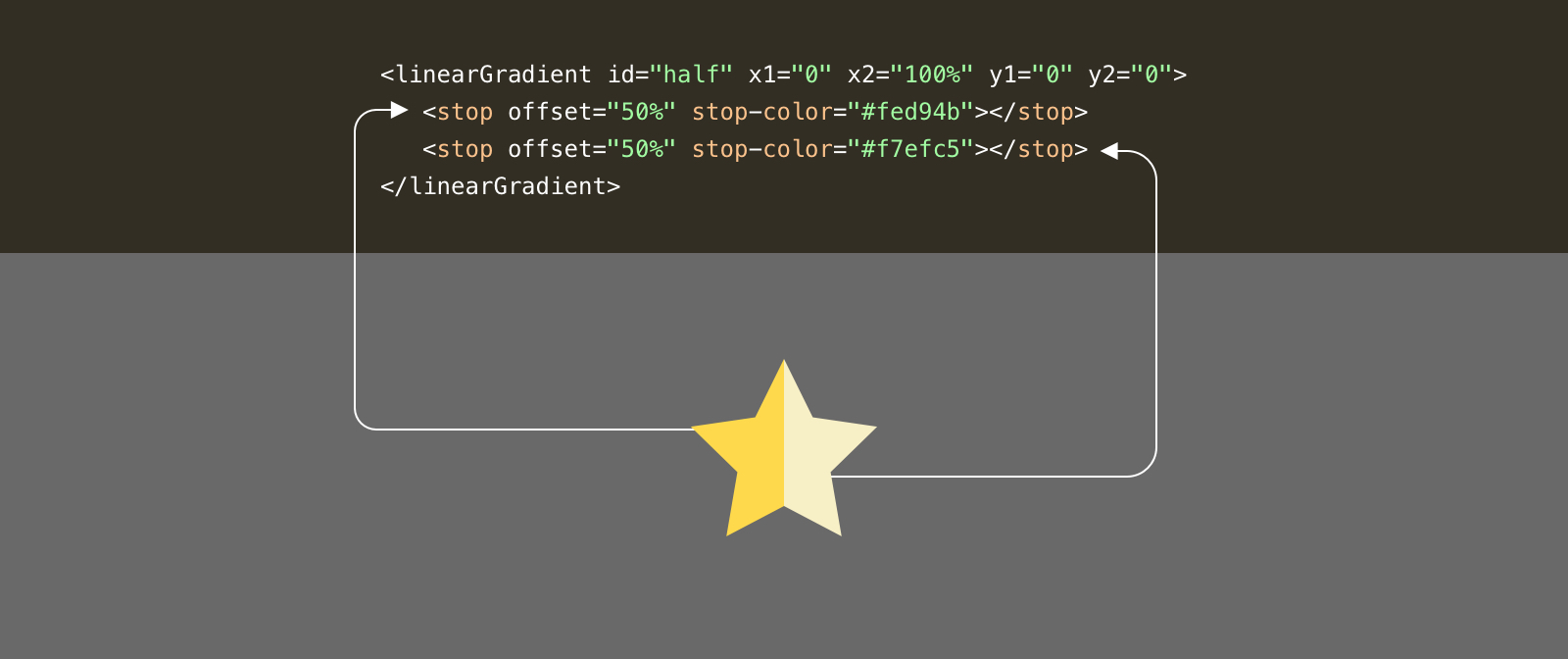Here is how we can use the gradient.

``````<p class="c-rate">
<svg class="c-icon" width="32" height="32" viewBox="0 0 32 32">
<use href="#star" fill="url(#half)"></use>
</svg>
</p>``````

Demo

### Outlined style

We want a variation that can be customized to include outlined stars. From a first glance, it seems simple. Let’s dig into the details.#### Outlined style for the SVG mask solution

To add a stroke, all we need is to add `stroke` to the SVG element. This will work fine for full stars. However, for partial ones, it will be cut-off because of the mask.To work around that, we need to have another star shape just for the outline. We can do that by duplicating the `<use>` element and removing the mask from it.

``````<p class="c-rate">
<svg class="c-icon" width="32" height="32" viewBox="0 0 32 32">
<use href="#star" fill="none" stroke="grey"></use>
</svg>
</p>``````

Notice that we have two `<use>` elements. The one with the mask, and the one with a stroke only. That’s how an outline style can be done with SVG masks.

Demo

#### Outlined style for the SVG gradient solution

For the gradient solution, we don’t need to duplicate the icon since there is no mask at all. What we need to do is to add a stroke and it’s done.

``````<svg style="width: 0; height: 0;" viewBox="0 0 32 32">
<defs>
<linearGradient id="half" x1="0" x2="100%" y1="0" y2="0">
<stop offset="50%" stop-color="#f7efc5"></stop>
<stop offset="50%" stop-color="#fed94b"></stop>
</defs>
</svg>

<p class="c-rate">
<svg class="c-icon" width="32" height="32" viewBox="0 0 32 32">
<use href="#star" fill="url(#half)" stroke="grey"></use>
</svg>
</p>``````

Demo

### Sizing

By using CSS variables and making sure that the SVG has the right `viewBox` attribute, we can easily size them.

``````.c-icon {
width: var(--size, 24px)
height: var(--size, 24px);
}

.c-icon--md {
--size: 40px;
}

.c-icon--lg {
--size: 64px;
}``````

I hope you enjoyed the article. Thanks for reading!